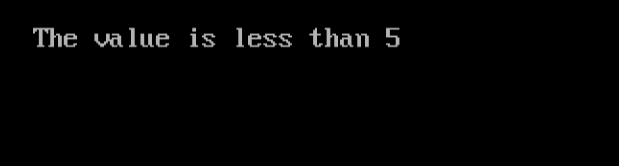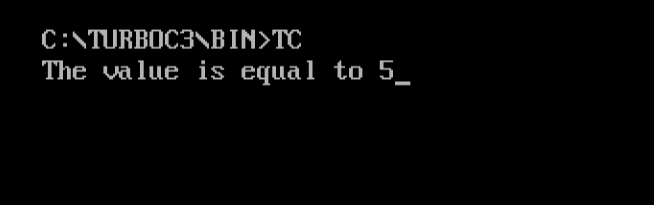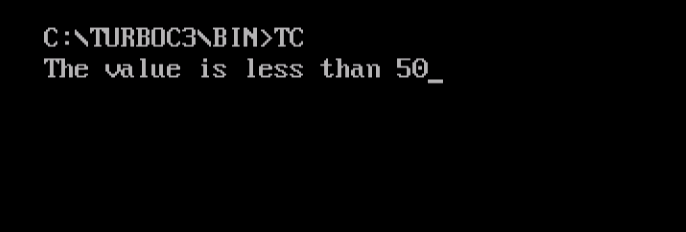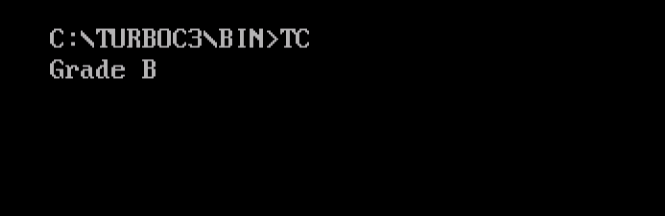# Decision Making Statements in C

## 12 Jan Decision Making Statements in C

Decision making statements in C language is the same as in other programming languages such as, C++, Java, etc. Decision making is needed when you want a set of instruction to be executed in a condition, and other set of instructions in another condition.

The following are the decision making statements in C,

## if  statement

If the condition is true, the statement gets executed. If the condition is false, it does nothing.

### Syntax

The following is the syntax for the if statement in C language,

The following syntax is for multiple statements,

The following is an example showing the usage of if statement in C language.

The following is the output, wherein if statement executes since the value of i is more than 5:## if-else statement

If the condition is true, then a set of statement is executed. If the condition is false, another set of statement gets executed.

### Syntax

The following is the syntax for if-else statement in C language,

The following is an example showing the usage of if-else statement in C language,

Here’s the output,You can also work upon nested if-elses. Let us see how we can do it for the preceding example,

The following is the output, showing what value gets displayed while using nested if-else,## else-if statement

The else-if statement is useful when if-statement has more than one condition.

### Syntax

The following is the syntax for else-if statement in C languages,

The following is an example showing the usage of else-if statement in C languages:

The following is the output, displaying the result for value less than 50,## Switch Statement

Use switch statement, if you want to take a decision from more than one choice. For example, grades A, B, C, D, E, etc based on marks of student.

The following is an example showing the usage of switch statement in C language,

The following is the output, displaying the result for 75 marks,In this lesson, we learned how to work with Decision Making Statements in C Programming. We saw how to implement if, if-else, else-if, and switch statements in C.We work to create free tutorials for all.